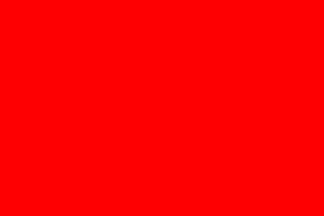## SVG

SVG或可缩放矢量图形是用于在网页中定义基于矢量的图形的Web标准。基于XML，SVG标准提供标记来描述视口中的路径，形状和文本。标记可以直接嵌入到HTML中以供显示，也可以保存到.svg文件中，并像任何其他图像一样插入。您可以手动编写SVG，但可以在矢量图形编辑器（如Illustrator或InkScape）中设计更复杂的图形，并将其导出为SVG文件或代码。

## SVG基础知识

<svg xmlns="http://www.w3.org/2000/svg" version="1.1">

</svg> 

<svg
version="1.1"
width="100%"
viewbox="0 0 600 300"
xmlns="http://www.w3.org/2000/svg">
<rect x="10" y="10" width="100" height="100" fill="#f7b2c1" />
<circle cx="240" cy="60" r="50" fill="#c1b2f7" stroke="#b2c1f7" stroke-width="15"/>
<text x="450" y="70" font-size="20" text-anchor="middle">SVG Text is browser readable!</text>
<g stroke="#b2c1f7"> <!-- g is for group -->
<path stroke-width="2" d="M10 170 l590 0" />
<path stroke-width="4" d="M10 190 l590 0" />
<path stroke-width="6" d="M10 210 l590 0" />
</g>
</svg> 

<path />元素定义视口中的矢量路径。路径由d属性定义。在第一个例子中，定义读取'移动到绝对坐标（10,170） 绘制一条线到X方向的相对坐标590和Y方向的0。

M =搬到 L =行到 H =水平线到 V =垂直线到 Z =关闭路径 C =（三次贝塞尔曲线）曲线 S =平滑曲线 Q =二次贝塞尔曲线到 T =平滑的二次贝塞尔曲线 A =弧

### 画布元素

<p > Before canvas . </p >
< canvas width ="120" height ="60" > </ canvas >
<p > After canvas . </p >
< script >
var canvas = document . querySelector (" canvas ") ;
var context = canvas . getContext ("2 d ") ;
context . fillStyle = " red ";
context . fillRect (10 , 10 , 100 , 50) ;
</ script >### 绘制饼图

results变量包含一个表示对象的对象数组 调查回复。

var results = [
{ name : " Satisfied " , count : 1043 , color : " lightblue "} ,
{ name : " Neutral " , count : 563 , color : " lightgreen "} ,
{ name : " Unsatisfied " , count : 510 , color : " pink "} ,
{ name : " No comment " , count : 175 , color : " silver "}
]; 

< canvas width ="200" height ="200" > </ canvas >
< script >
var cx = document . querySelector (" canvas ") . getContext ("2 d ") ;
var total = results . reduce ( function ( sum , choice ) {
return sum + choice . count ;
} , 0) ;

// Start at the top

var currentAngle = -0.5 * Math . PI ;
results . forEach ( function ( result ) {
var sliceAngle = ( result . count / total ) * 2 * Math . PI ;
cx . beginPath () ;
// center =100 ,100 , radius =100
// from current angle , clockwise by slice ' s angle
cx . arc (100 , 100 , 100 ,
currentAngle , currentAngle + sliceAngle );
currentAngle += sliceAngle ;
cx . lineTo (100 , 100) ;
cx . fillStyle = result . color ;
cx . fill () ;
}) ;
</ script > 

## 编者

• Vectr - 免费提供和创建和编辑SVG图形的网络和桌面工具

## 用于创建SVG的工具

• Inkscape - 它是一个开源工具，用于使用易于使用的图形界面进行最先进的矢量绘图。